# Mountain railway

Height difference between points A, B of railway line is 38.5 meters, their horizontal distance is 3.5 km. Determine average climb in permille up the track.

Result

x =  11

#### Solution:Leave us a comment of example and its solution (i.e. if it is still somewhat unclear...):

Showing 0 comments:Be the first to comment!#### To solve this example are needed these knowledge from mathematics:

Our permille calculator will help you quickly calculate various typical tasks with permilles. Do you want to convert length units? See also our right triangle calculator. See also our trigonometric triangle calculator. Try conversion angle units angle degrees, minutes, seconds, radians, grads.

## Next similar examples:

1. Two municipalities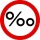The horizontal distance between municipalities is 39 km. Average sinking is 7 per mille. What is the difference in height between these municipalities?
2. Obtuse angle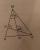The line OH is the height of the triangle DOM, line MN is the bisector of angle DMO. obtuse angle between the lines MN and OH is four times larger than the angle DMN. What size is the angle DMO? (see attached image)
3. Mirror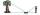How far must Paul place a mirror to see the top of the tower 12 m high? The height of Paul's eyes above the horizontal plane is 160 cm and Paul is from the tower distant 20 m.
4. StairwayStairway has 20 steps. Each step has a length of 22 cm and a height of 15 cm. Calculate the length of the handrail of staircases if on the top and bottom exceeds 10 cm.
5. Plane IIA plane flew 50 km on a bearing 63degrees20 and the flew on a bearing 153degrees20 for 140km. Find the distance between the starting point and the ending point
6. Plan scaleAt what scale is drawn plan of the building, where one side of the building is 45 meters long is on the plan expressed by a straight line 12 mm long.
7. Road - permille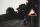5 km long road begins at an altitude 500 meters above sea level and ends at a altitude 521 ASL. How many permille road rises?
8. Obtuse angleWhich obtuse angle is creating clocks at 17:00?
9. Feet to milesA student runs 2640 feet. If the student runs an additional 7920 feet, how many total miles does the student run?
10. Center traverseIt is true that the middle traverse bisects the triangle?
11. Degrees to radiansConvert magnitude of the angle α = 9°39'15" to radians:
12. AnglesThe outer angle of the triangle ABC at the vertex A is 114°12'. The outer angle at the vertex B is 139°18'. What size is the internal angle at the vertex C?
13. ClimbFor horizontal distance 4.9 km road rise by 6.1 m. Calculate the road pitch in ‰ (permille, parts per thousand).
14. Outer anglesThe outer angle of the triangle ABC at the A vertex is 71°40 ' outer angle at the vertx B is 136°50'. What size has the inner triangle angle at the vertex C?
15. Complementary angles 2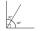Two complementary angles are (x+4) and (2x - 7) find the value of x
16. Right triangleRight triangle ABC with side a = 19 and the area S = 95. Calculate the length of the remaining sides.
17. 30-60-90The longer leg of a 30°-60°-90° triangle measures 5. What is the length of the shorter leg?